## Efficient Rendering of Layered Materialsusing an Atomic Decomposition with Statistical Operators

Laurent Belcour## Last Year ...

Rendered in Unity

## Last Year ...

• Rendering thin-film iridescence
• Using a clear-coat plugin in Mitsuba
• But no clear-coat available in Unity 😭
• I wanted to show the beetle live
• One solution: code one in time!
• Turn out we can do much more
• Multiple rough interfaces
• Energy conservation
• ...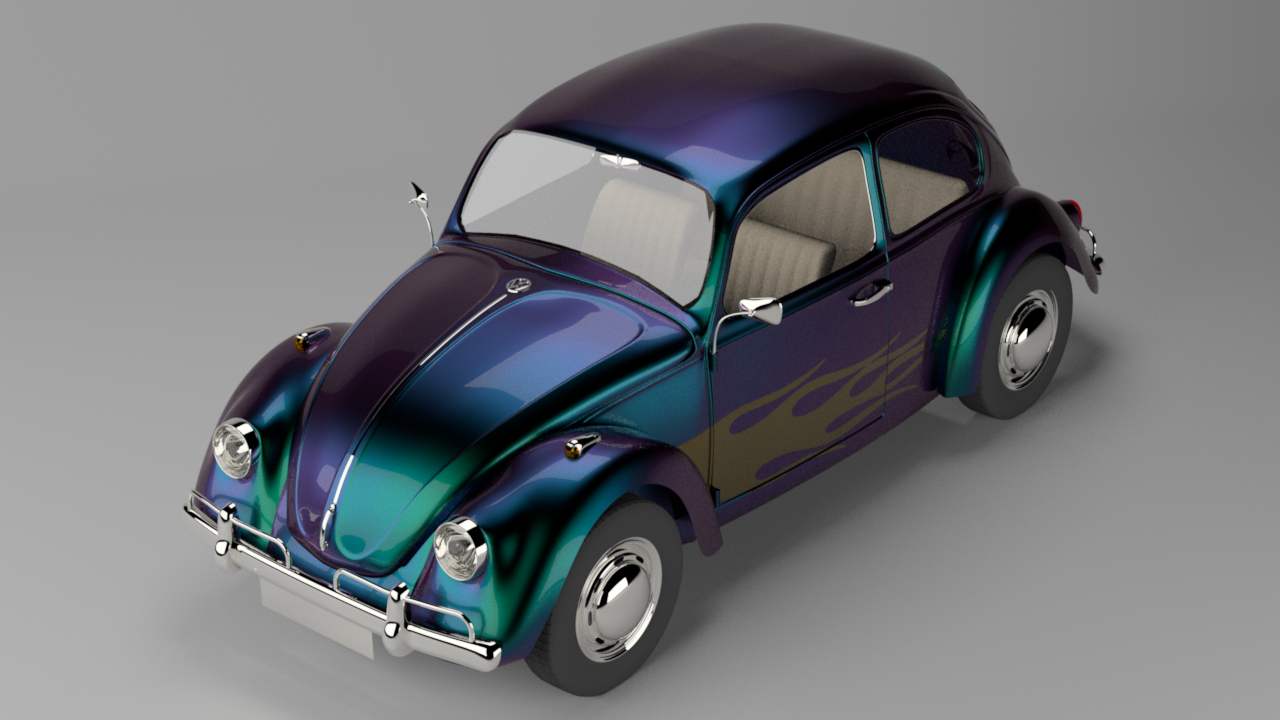Rendered in Mitsuba## Layered Materials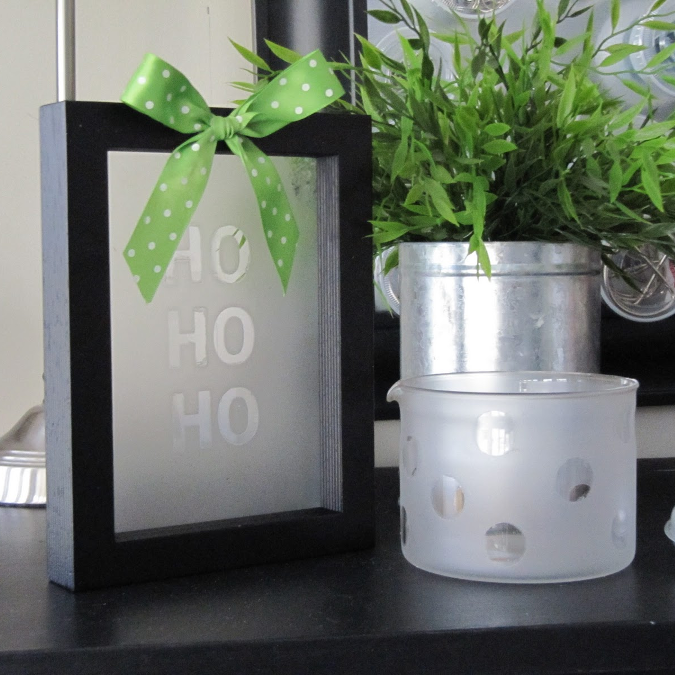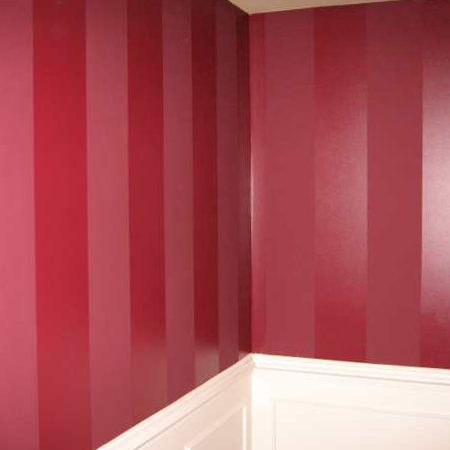## Layered Materials

$\boldsymbol{\omega}_i$
$\boldsymbol{\omega}_o$

## Layered Materials

• Brute-force rendering is not possible
• At least not in real-time graphics
• Precomputation is not statisfactory
• Forbid to use textured assets
• Memory limitation on GPUs
• Our solution: tight approximation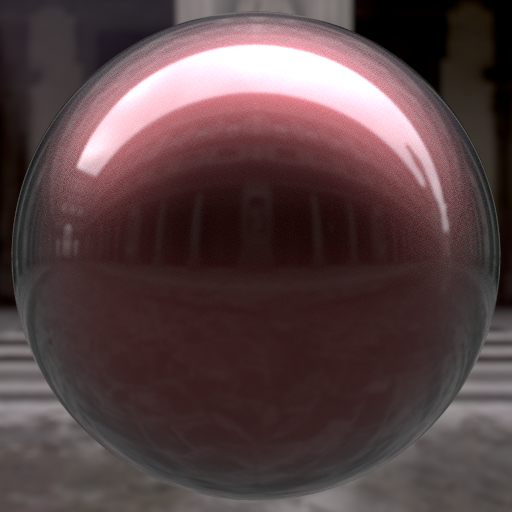LayerLab data - 1.7GiB [Jakob 2014]

## Our Idea: Sum of GGX Lobes

$\mathbf{\omega}_i$
$\mathbf{n}$
$$\rho(\mathbf{\omega}_i, \mathbf{\omega}_o) = \sum_{k} w_k \, \rho_k(\mathbf{\omega}_i, \mathbf{\omega}_o)$$

## Statistical Analysis: Mapping• We study BSDF statistics
• In the orthographicaly projected disc
• There, GGX is almost rotationaly symmetric
Orthographic projection
GGX lobe with $\alpha = 0.01$

## Statistical Analysis: Mapping

• We study BSDF statistics
• In the orthographicaly projected disc
• There, GGX is almost rotationaly symmetric
• To find a mapping
• From the three moments (energy, mean, variance)
• To a BRDF lobe parameters (albedo, view, roughness)
Orthographic projection
Equivalent Statistics
$\mathbf{\omega}_i$
$(e, \mathbf{\mu}, \sigma)$

## Statistical Analysis: Mapping

• We study BSDF statistics
• In the orthographicaly projected disc
• There, GGX is almost rotationaly symmetric
• To find a mapping
• From the three moments (energy, mean, variance)
• To a BRDF lobe parameters (albedo, view, roughness)
• Can we find the statistics of layered materials?

Layered configuration

## Statistical Analysis: Framework

• Infer statistics atomically
• Details in the paper
• Update $e$, $\mu$, and $\sigma$
• Example: refraction operator
• Shift, scales and convolves the incident lobe

$$e_t = \tilde{\mbox{F}} \, e_i$$

$$\mu_t = - \eta_{12} \, \mu_i$$

$$\sigma_t = \eta_{12} \, \color{blue}{\sigma_i} + \color{red}{s}$$

Reflection
Refraction
Scattering

## Statistical Analysis: Validation

• Interactively testing atomic operators

## Statistical Analysis: Framework

• Multiple layers: chain operators

## Statistical Analysis: Framework

• Merging statistics
$(e_1, \mu_1, \sigma_1)$
$(e, \mu, \sigma)$
$(e, \mu, \sigma) = \left(e_1+e_2, \mu, \dfrac{e_1}{e}\sigma_1+\dfrac{e_2}{e}\sigma_2\right)$
$(e_2, \mu_2, \sigma_2)$
$+$

## Statistical Analysis: Framework

• Merging statistics
• Multiple scattering
• Closed-form statistics
• Arithmetico-Geometric series

## Statistical Analysis: Framework

• Merging statistics
• Multiple scattering
• Closed-form statistics
• Arithmetico-Geometric series

## Offline Validation

• Mitsuba renderer
• Both opaque and transparent plugins
• Varying number of textured layers
• Multiple Importance Sampling with the lobes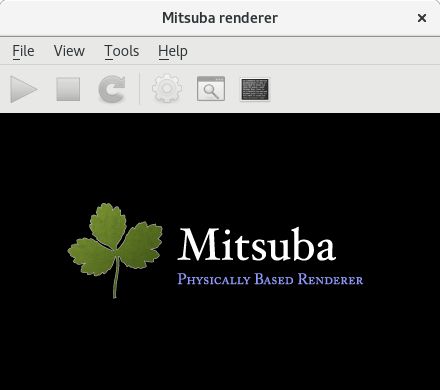## Offline Validation

• Mitsuba renderer
• Comparison with stochastic reference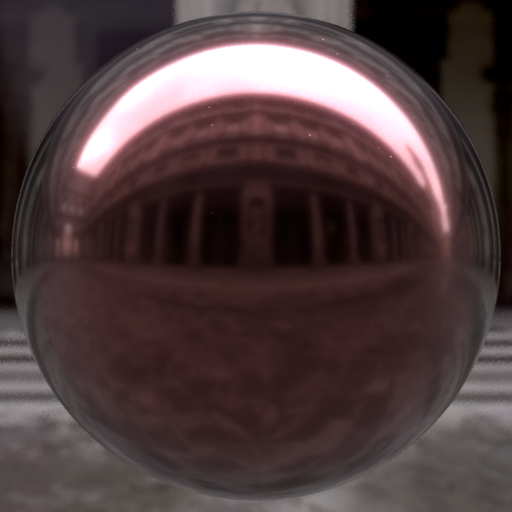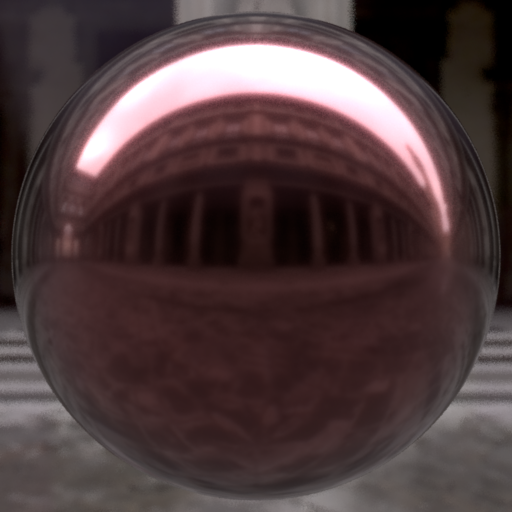Ours Reference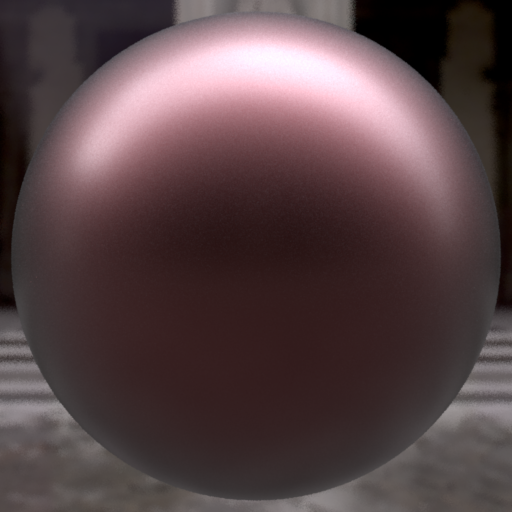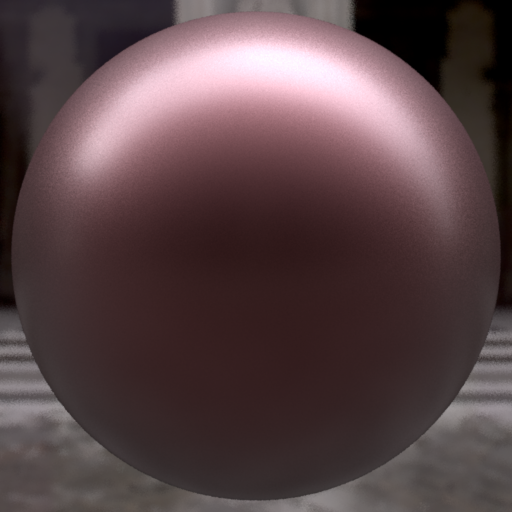Ours Reference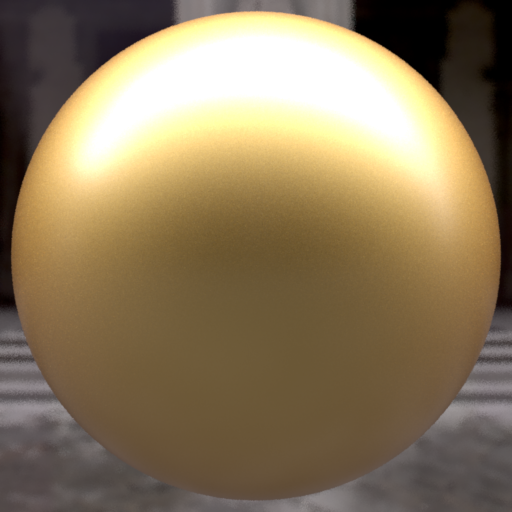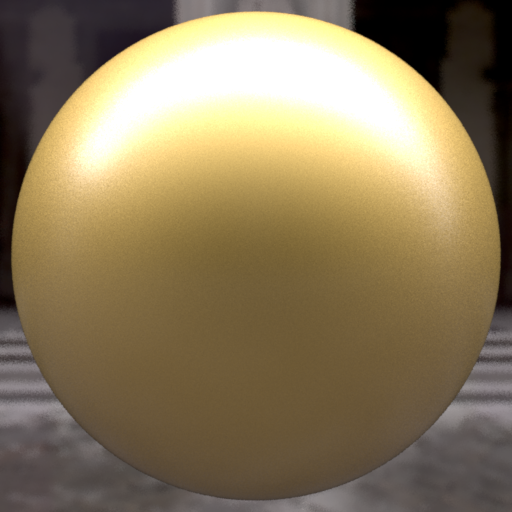Ours Reference Metal foil Rough metal Gold Coated

## Offline Validation

• Mitsuba renderer
• Comparison with stochastic reference
• Layered method of Weidlich & Wilkie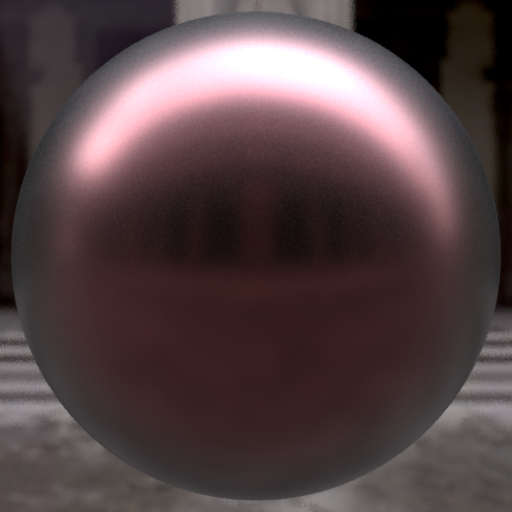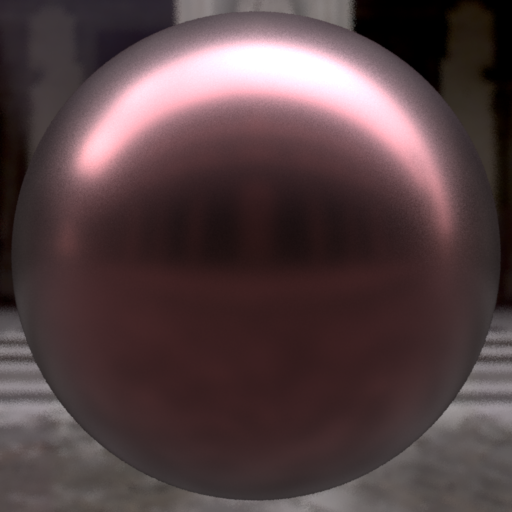Ours
Reference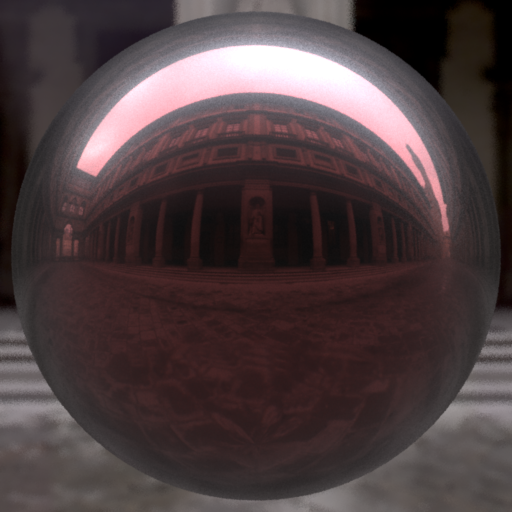[WW07]
Reference

## Offline Validation

• Mitsuba renderer
• Comparison with stochastic reference
• Layered method of Weidlich & Wilkie 
• Multiple scattering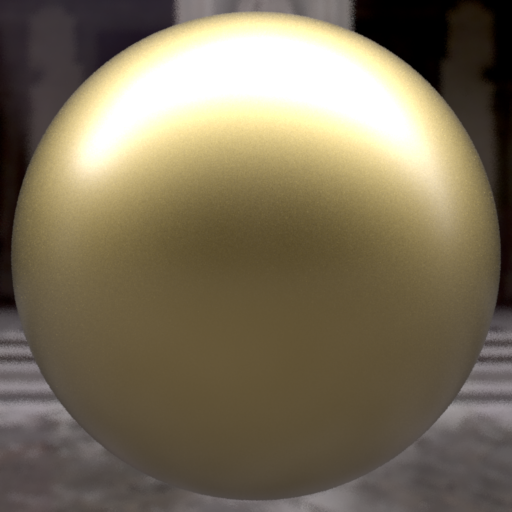$R + TRT$ $R + TR^+T$ Ours

## Offline Results: Textures

 Textured base $\alpha$ Textured top $\eta$ Textured top $\alpha$

## Offline Results: Robot Bust

• Two layer configuration
• Multiple textured layers
• Base and top Index of Refraction
• Top roughness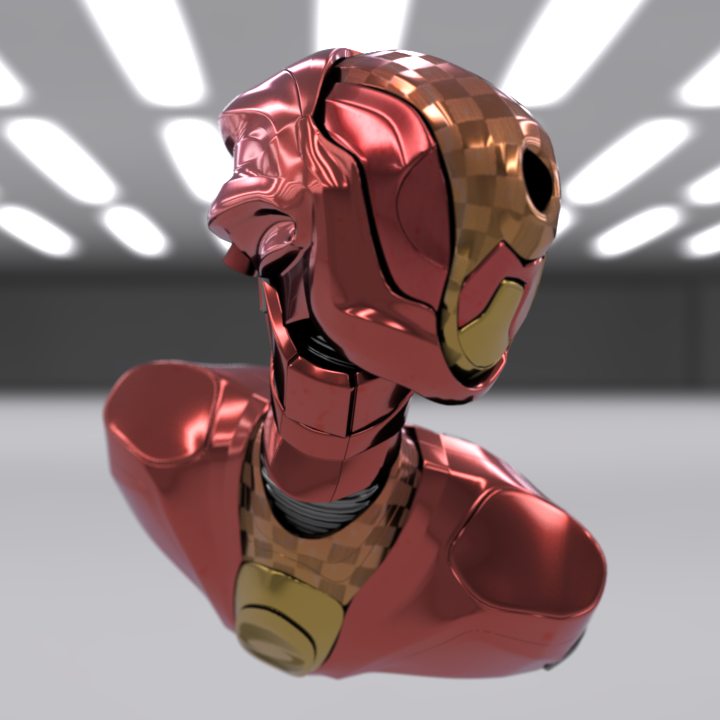## Offline Results: Dragon

• Gold metal dragon
• Simulate dust
• Increase the haze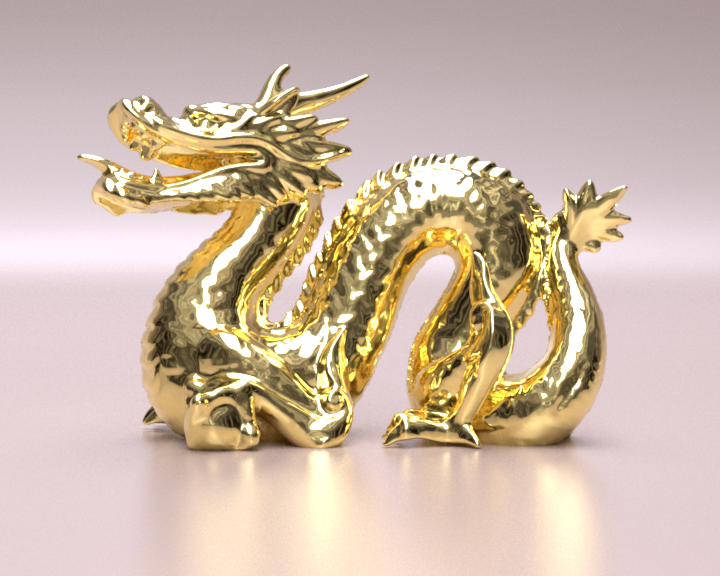## Limitations: High Roughnesses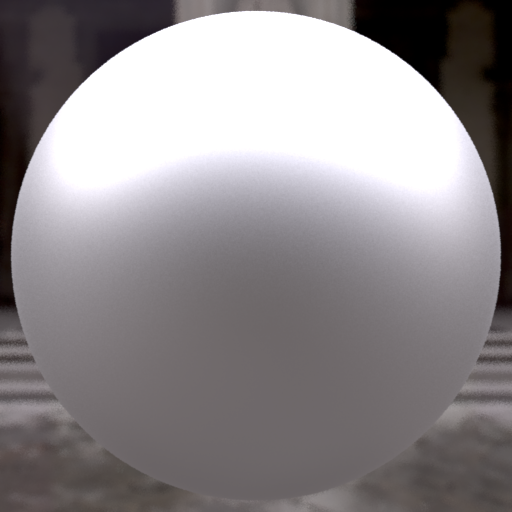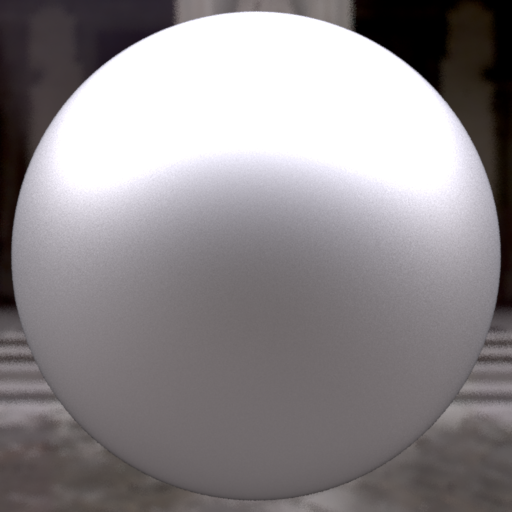$\alpha = 0.3$
Ours
Reference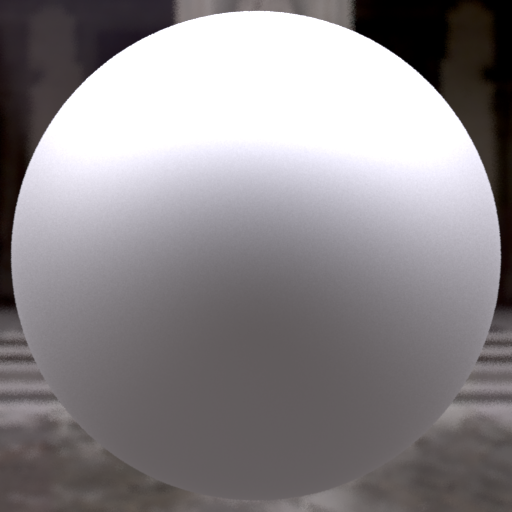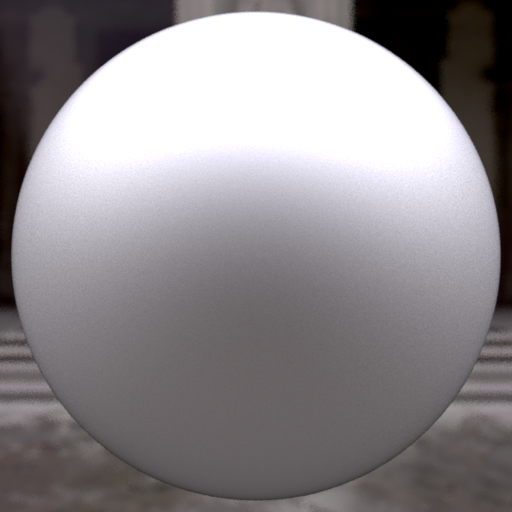$\alpha = 0.6$
Ours
Reference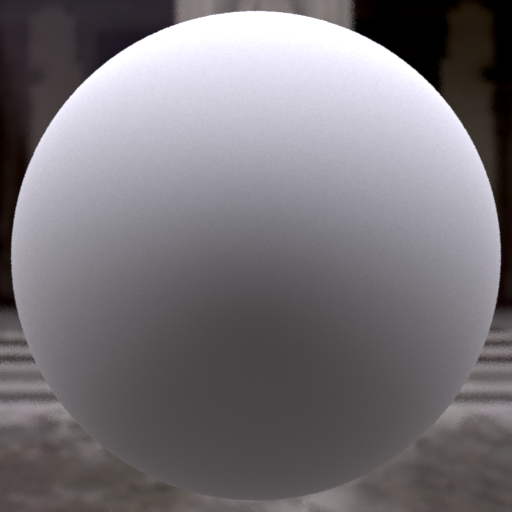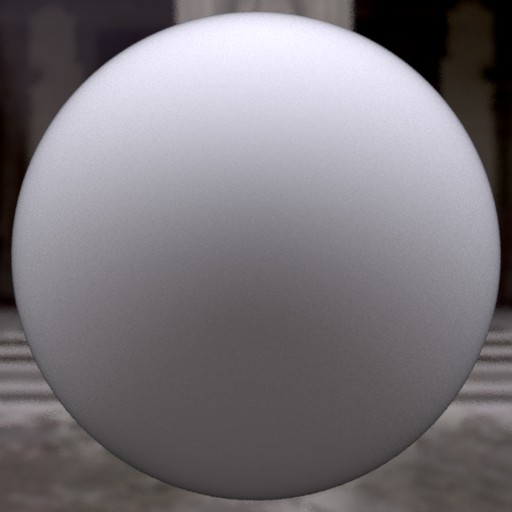$\alpha = 0.9$
Ours
Reference

## Summary

• A novel BSDF model for layered structures
• Accurate for low roughnesses
• Accounts from multiple scattering
• No parameter dependent precomputation
• Compatible with real-time scenario
• Our contributions
• Statistical analysis of light transport in layers
• New adding-doubling scheme for variance

## Thank you for your attention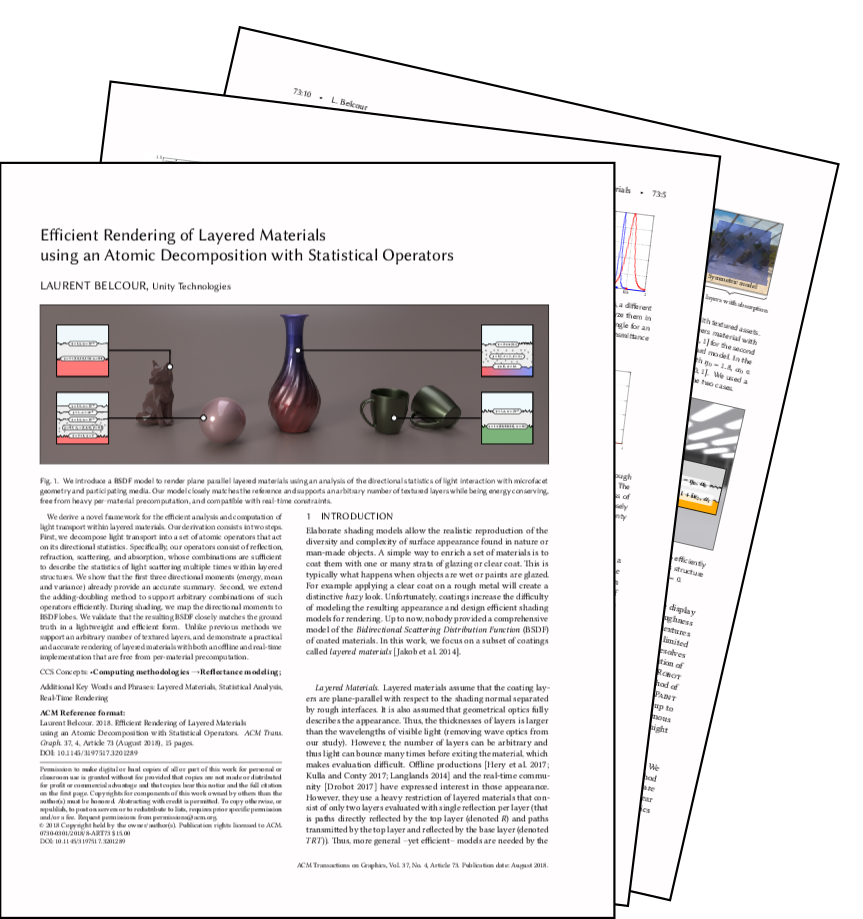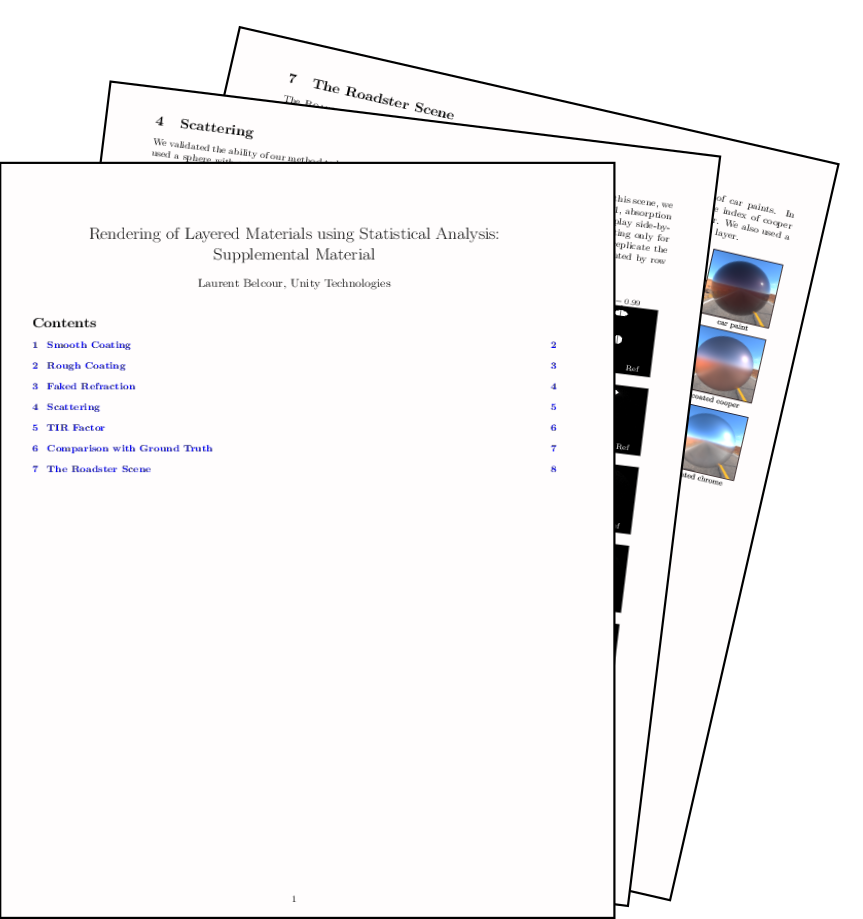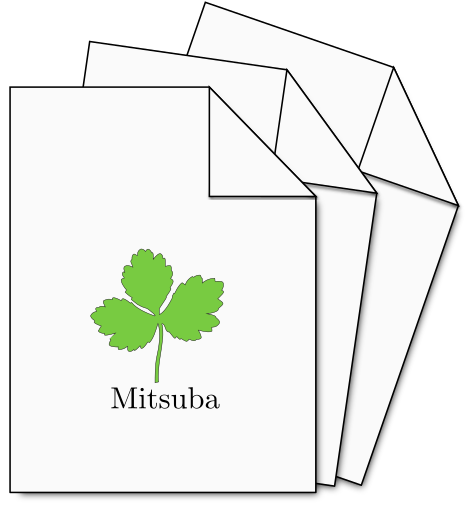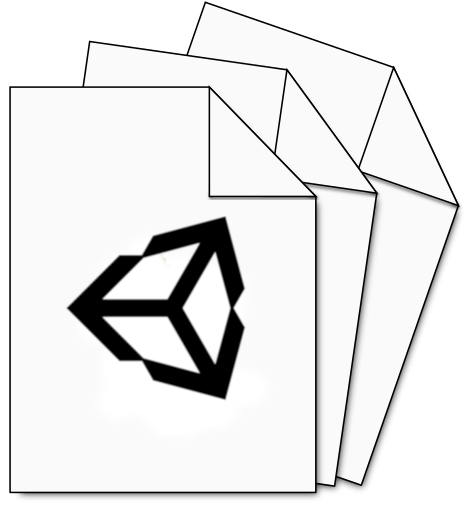paper supp. mat. code HDRP StackLit
available at belcour.github.io/blog